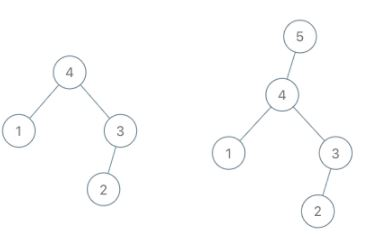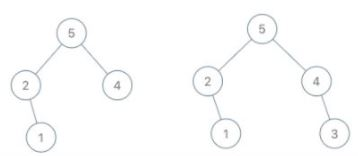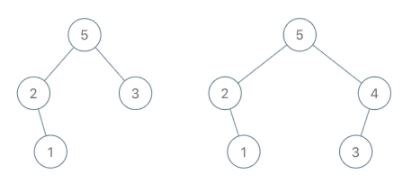## Algorithm

Problem Name: 998. Maximum Binary Tree II

A maximum tree is a tree where every node has a value greater than any other value in its subtree.

You are given the `root` of a maximum binary tree and an integer `val`.

Just as in the previous problem, the given tree was constructed from a list `a` (`root = Construct(a)`) recursively with the following `Construct(a)` routine:

• If `a` is empty, return `null`.
• Otherwise, let `a[i]` be the largest element of `a`. Create a `root` node with the value `a[i]`.
• The left child of `root` will be `Construct([a, a, ..., a[i - 1]])`.
• The right child of `root` will be `Construct([a[i + 1], a[i + 2], ..., a[a.length - 1]])`.
• Return `root`.

Note that we were not given `a` directly, only a root node `root = Construct(a)`.

Suppose `b` is a copy of `a` with the value `val` appended to it. It is guaranteed that `b` has unique values.

Return `Construct(b)`.

Example 1:```Input: root = [4,1,3,null,null,2], val = 5
Output: [5,4,null,1,3,null,null,2]
Explanation: a = [1,4,2,3], b = [1,4,2,3,5]
```

Example 2:```Input: root = [5,2,4,null,1], val = 3
Output: [5,2,4,null,1,null,3]
Explanation: a = [2,1,5,4], b = [2,1,5,4,3]
```

Example 3:```Input: root = [5,2,3,null,1], val = 4
Output: [5,2,4,null,1,3]
Explanation: a = [2,1,5,3], b = [2,1,5,3,4]
```

Constraints:

• The number of nodes in the tree is in the range `[1, 100]`.
• `1 <= Node.val <= 100`
• All the values of the tree are unique.
• `1 <= val <= 100`

## Code Examples

### #1 Code Example with Java Programming

```Code - Java Programming```

``````
class Solution {
public TreeNode insertIntoMaxTree(TreeNode root, int val) {
if(root == null) {
return new TreeNode(val);
}

if(root.val < val){
TreeNode node = new TreeNode(val);
node.left = root;
return node;
}

root.right = insertIntoMaxTree(root.right, val);

return root;
}
}
``````
Copy The Code &

Input

cmd
root = [4,1,3,null,null,2], val = 5

Output

cmd
[5,4,null,1,3,null,null,2]

### #2 Code Example with Python Programming

```Code - Python Programming```

``````
class Solution:
def insertIntoMaxTree(self, root: TreeNode, val: int, parent = None) -> TreeNode:
if not root or val > root.val:
new = TreeNode(val)
new.left = root
if parent:
if parent.right == root:
parent.right = new
else:
parent.left = new
root = new
else:
root.right = self.insertIntoMaxTree(root.right, val, root)
return root
``````
Copy The Code &

Input

cmd
root = [4,1,3,null,null,2], val = 5

Output

cmd
[5,4,null,1,3,null,null,2]

### #3 Code Example with C# Programming

```Code - C# Programming```

``````
namespace LeetCode
{
public class _0998_MaximumBinaryTreeII
{
public TreeNode InsertIntoMaxTree(TreeNode root, int val)
{
var newNode = new TreeNode(val);
TreeNode prev = null, curr = root;

while (curr != null && curr.val > newNode.val)
{
prev = curr;
curr = curr.right;
}

if (prev != null)
prev.right = newNode;
else
root = newNode;

if (curr != null)
newNode.left = curr;

return root;
}
}
}
``````
Copy The Code &

Input

cmd
root = [5,2,4,null,1], val = 3

Output

cmd
[5,2,4,null,1,null,3]